# Multiplying and Dividing Scientific Notation Lesson Plan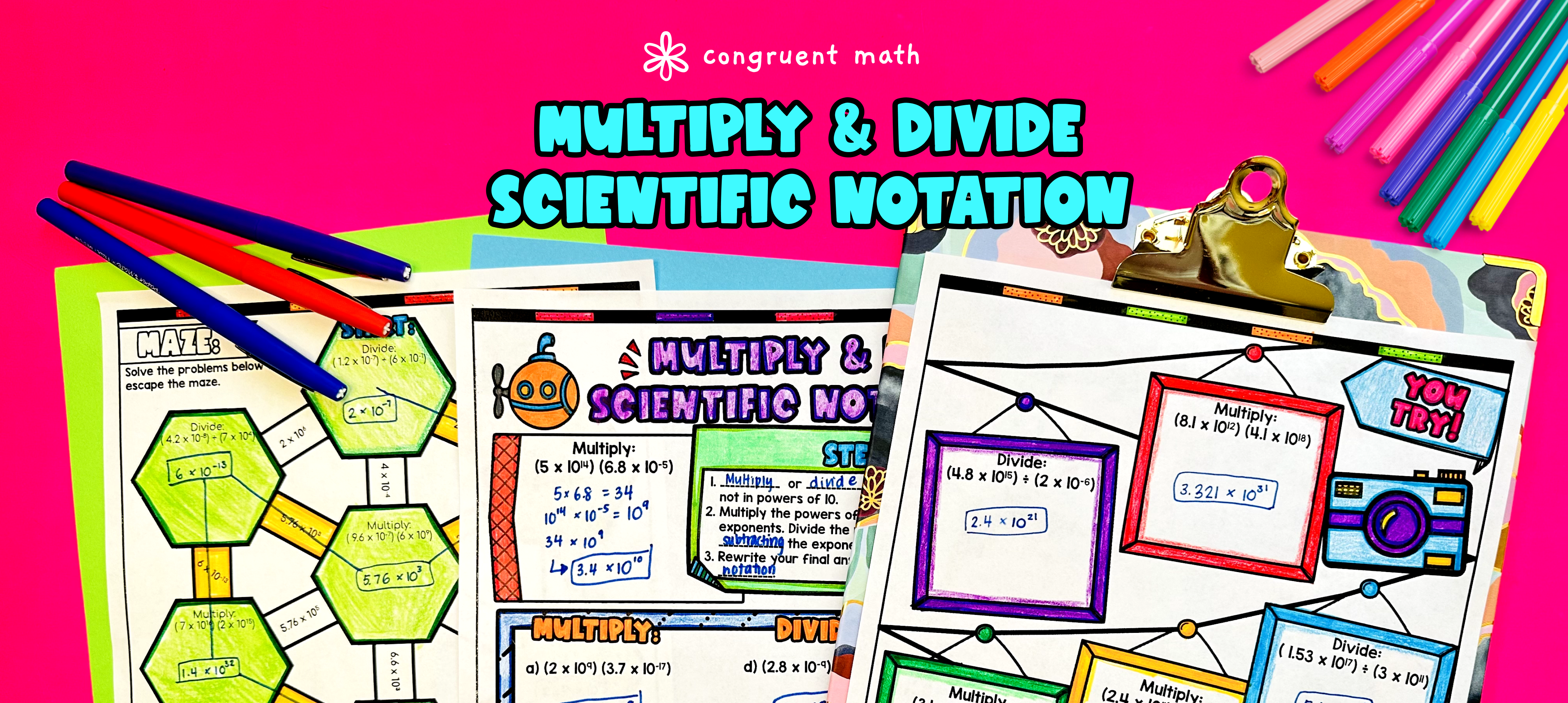Pin This

## Overview

Ever wondered how to teach multiplying and dividing numbers in scientific notation in an engaging way to your eighth-grade students?

In this lesson plan, students will learn about scientific notation and its real-life applications. Through artistic, interactive guided notes, maze activity, a doodle & color by number activity, and a maze worksheet, students will gain a comprehensive understanding of multiplying and dividing numbers in scientific notation.

The lesson culminates with a real-life application activity where students read and reflect about real-life uses of scientific notation.

## Get the Lesson Materials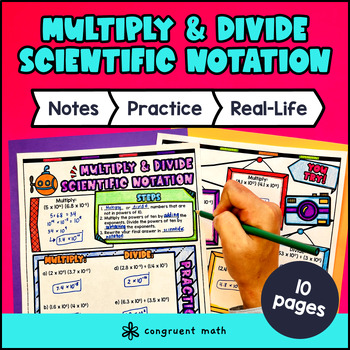\$4.25

## Learning Objectives

After this lesson, students will be able to:

• Multiply numbers expressed in scientific notation

• Divide numbers expressed in scientific notation

• Apply scientific notation to solve real-life problems and situations

• Recognize the importance and relevance of using scientific notation in various fields of study

## Prerequisites

Before this lesson, students should be familiar with:

• Basic operations with decimals and integers

• Understanding of exponents and powers of 10

## Key Vocabulary

• Scientific notation

• Exponent

• Base

• Coefficient

## Procedure

### Introduction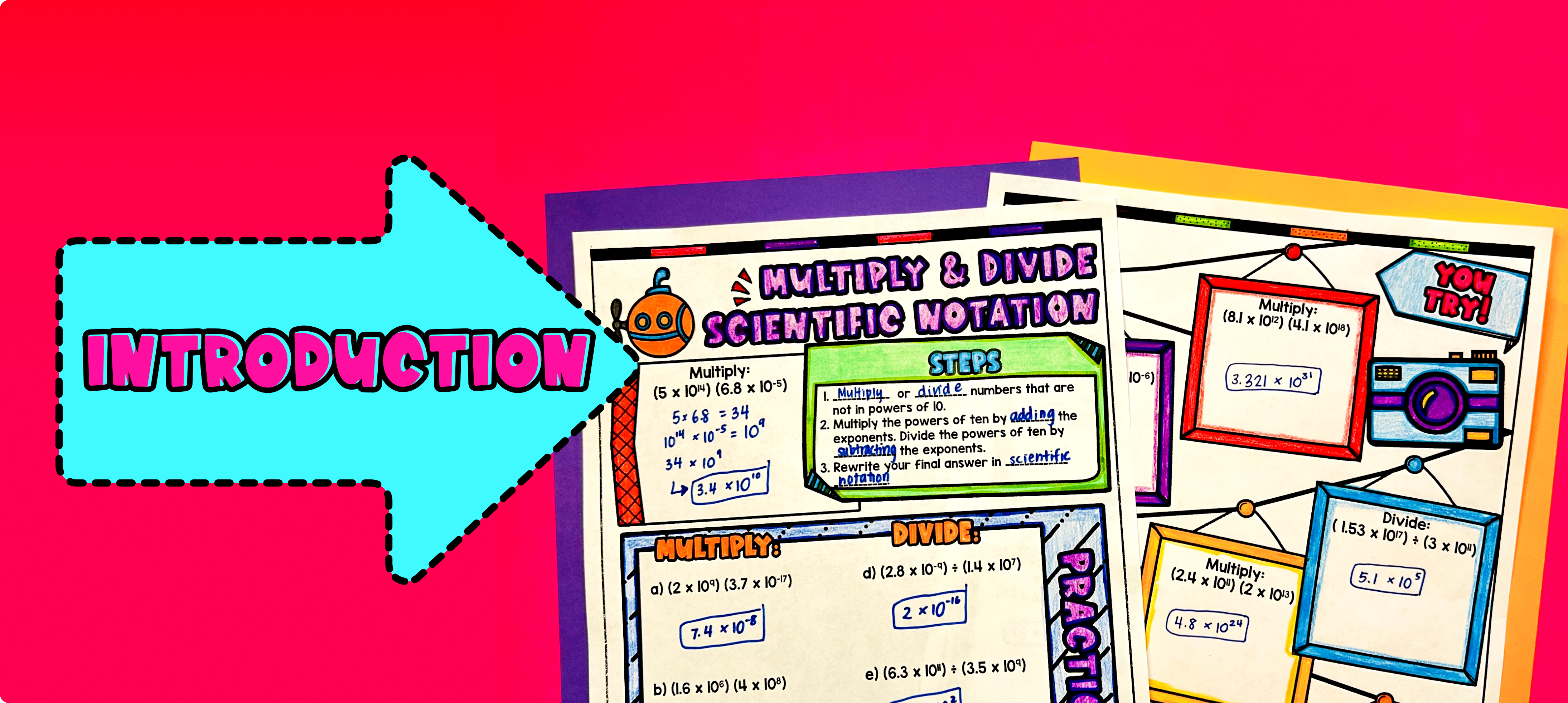Pin This

As a hook, ask students why scientific notation is important in real life situations. You can prompt them to think about situations where very large or very small numbers are involved, such as the distance between planets, the weight of atoms, or the population of cities. Refer to the real-life application section on the last page of the guided notes as well as the FAQs below for ideas.

Use the first page of the guided notes to introduce the concept of multiplying and dividing numbers in scientific notation. Walk through the key points of multiplying numbers in scientific notation, including how to multiply the coefficients and add the exponents.

Based on student responses, reteach the concept of multiplying numbers in scientific notation that students may need extra help with. If your class has a wide range of proficiency levels, you can pull out students for reteaching, and have more advanced students begin work on the practice exercises.

### Practice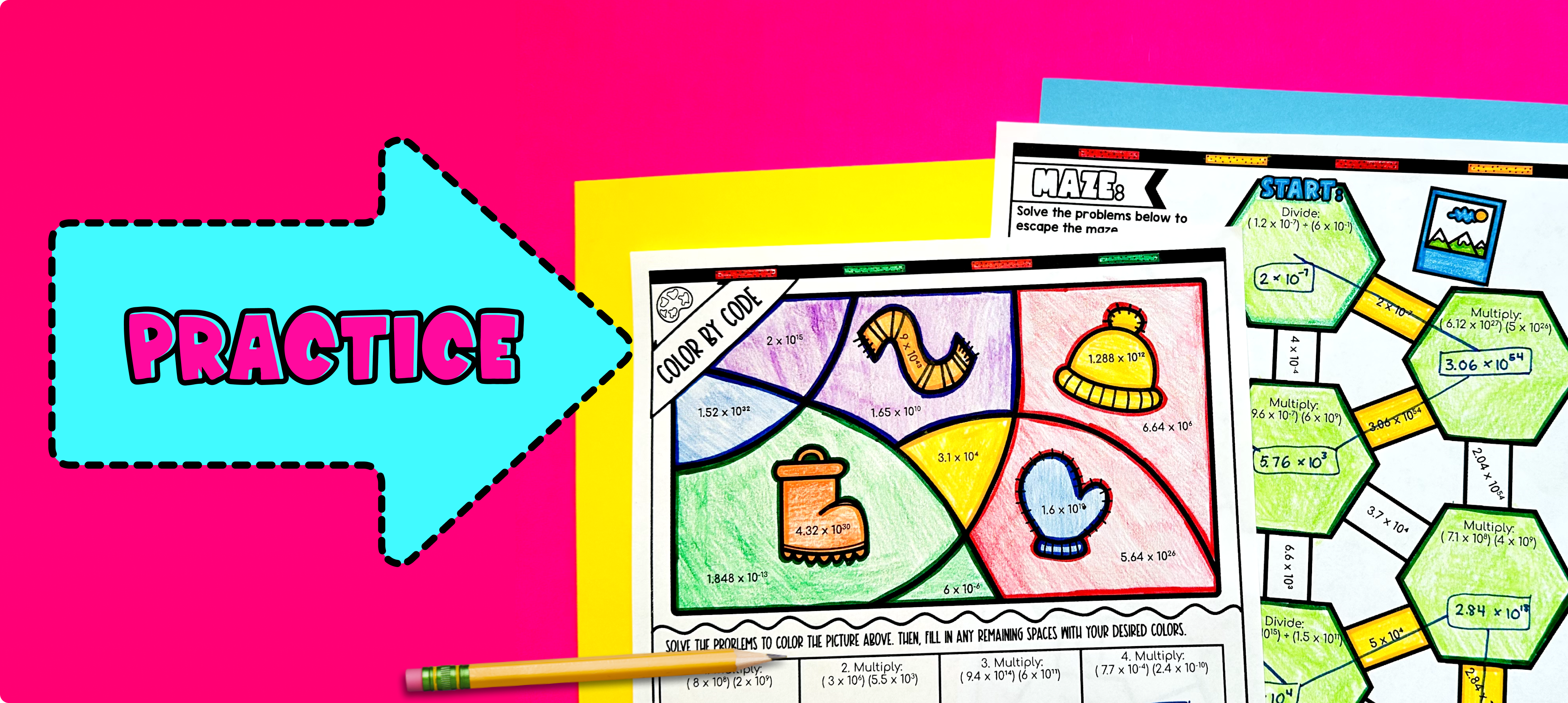Pin This

Have students practice multiplying and dividing numbers in scientific notation using the practice worksheets (maze and color by number activity in the guided notes). Walk around to answer student questions.

### Real-Life Application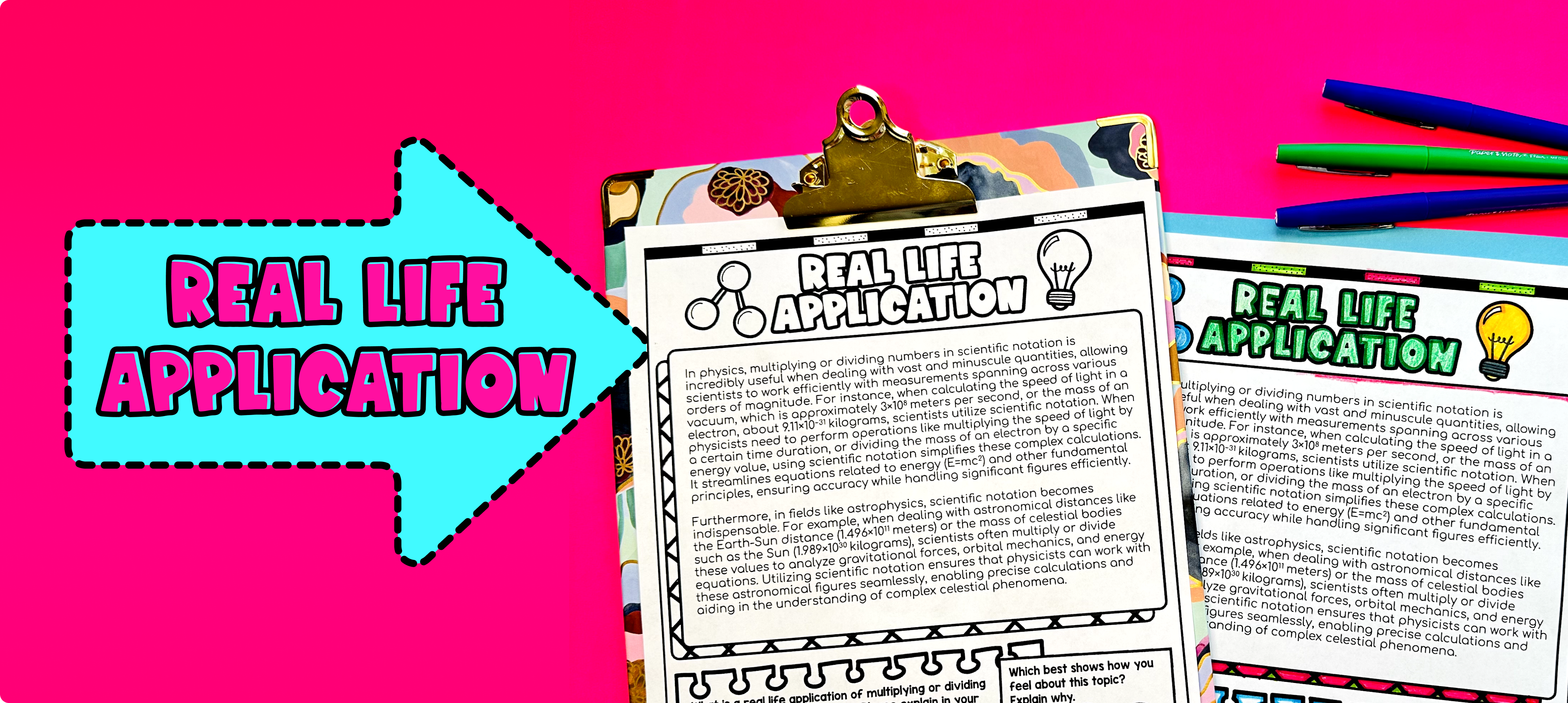Pin This

Bring the class back together, and introduce the concept of real-life uses of scientific notations. Explain that scientific notation is commonly used in scientific fields such as astronomy, physics, and chemistry. It is a way to express very large or very small numbers in a more convenient and concise format.

## Extensions

If you’re looking for digital practice for multiplying and dividing numbers in scientific notation, try my Pixel Art activities in Google Sheets. Every answer is automatically checked, and correct answers unlock parts of a mystery picture. It’s incredibly fun, and a powerful tool for differentiation.

Here are activities to explore: